# Molecular Device¶

Version: 2016.3

This tutorial focuses on the calculation and analysis of a molecular device. The device consists of a dithiol benzene (DTB) molecule (sometimes also called benzene dithiol, BDT) in contact with two gold (111) surfaces. This is a classic molecular device, sometimes called the fruit fly of molecular electronics, and it has been studied in a large number of publications, see for instance [STB+03][TJ05] and references therein.

Some of the calculations in this tutorial are quite time-consuming; for a quicker introduction to quantum transport studies with ATK, see Transport calculations with QuantumATK. The example structure you will use is a molecular junction with a benzene ring between two gold electrodes. You can find the instruction of how to build it in Molecular Junction.

The DFT model in QuantumATK is the underlying calculation engine used in this tutorial. A complete description of all the parameters, and in many cases a longer discussion about their physical relevance, can be found in the ATK reference manual.## Zero-bias calculation¶

Following the instruction of Molecular Junction, or download the script builder_device.py, you will get the following structure.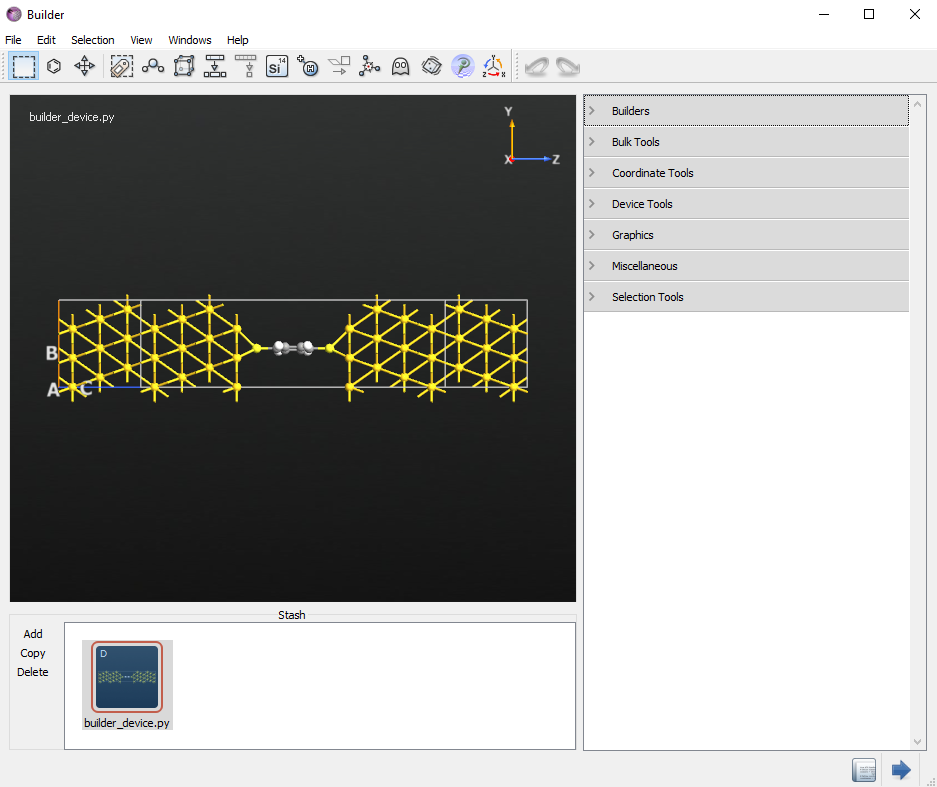Attention

The geometry was not relaxed. This is not critical for the concepts we will discuss in this tutorial, but it is an important step to consider for any real calculations with ATK. To learn how to relax a device geometry, see the Relaxation of devices using the OptimizeDeviceConfiguration study object.

### Setting up the calculation with the Script Generator¶

Now send the geometry to the Script Generator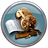by pressing the Send to button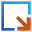at the lower right-hand corner of the Builder and select Script Generator from the pop-up menu.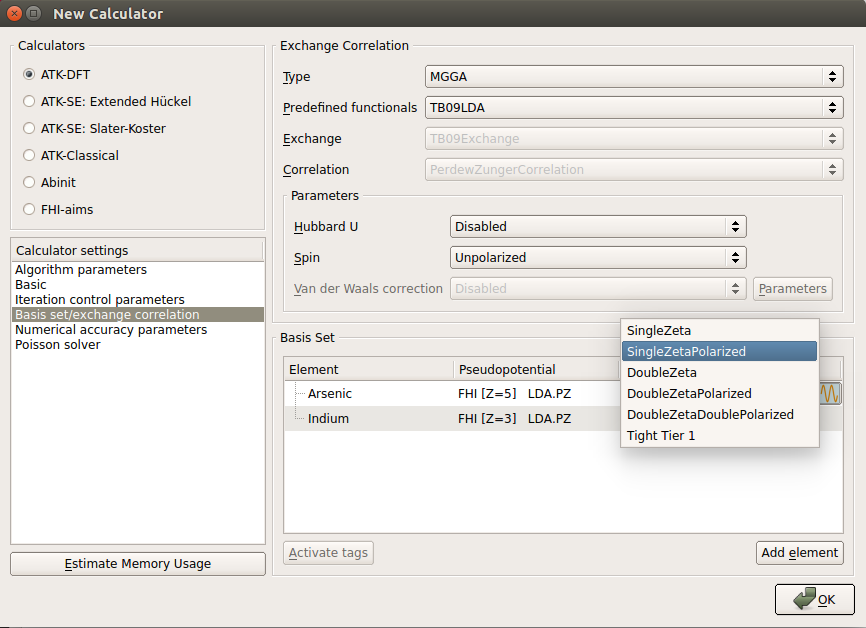To run the script on your local machine, send it to the Job Manager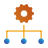by pressing the Send to buttonat the lower right-hand corner of the Script Generator and select Job Manager from the pop-up menu. In the Job Manager, press Run Queue to launch the job. On a modern, single-node computer the script should take around an hour to execute.

Note

The calculation speed can be increased greatly by using a parallel computer. For information about how to run jobs in parallel, see the Parallelization of QuantumATK calculations.

## Analysis of the zero-bias results¶

In this chapter you will extract data from the zero-bias calculation. You will do the following analyses:

• Compare the transmission spectrum with the Partial Device Density Of States (PDDOS) of the phenylene ring.
• Calculate the Molecular Projected Self-Consistent Hamiltonian (MPSH), and rationalize the transmission in terms of the MPSH states.
• Calculate the transmission eigenstates.
• Calculate the energy-dependent Local Density of States (LDOS), in order to obtain a spatial view of the energy levels.

### The transmission spectrum and density of states¶

Return to the main QuantumATK window. The au-dtb-au.hdf5 file should now be visible under Project Files, and its contents displayed on the LabFloor. It contains three objects:Select the TransmissionSpectrum object, and click the Transmission Analyzer in the right-hand panel bar.

The Transmission Analyzer plugin opens. On the left, named Spectrum, is shown the k-point averaged transmission spectrum as function of energy. The average Fermi energy of the two electrodes is set to zero and indicated with a dashed line.Fig. 82 The k-point averaged transmission spectrum as function of energy

The transmission and energy values in the point marked by the red dot are shown below the plot. You can use the mouse to move the dot along the $$T(E)$$ curve and read off the values. The Curves drop-down menu lets you select the spin components to be plotted. Note that the transmission for spin types X, Y, and Z are only non-zero for calculations with noncollinear spin.

At any particular energy (defined by the red dot), the total transmission is computed as an average over the transmission coefficients in the sampled k-points. The right-hand plot, named Coefficients, shows an interpolated contour plot of the transmission coefficients vs. reciprocal vectors $$k_A$$ and $$k_B$$. For example, at the energy, E = -1.4 eV, where you find a spectrum peak at the transmission, the contribution is minimum around the $$\Gamma$$ point, as shown in the figure below.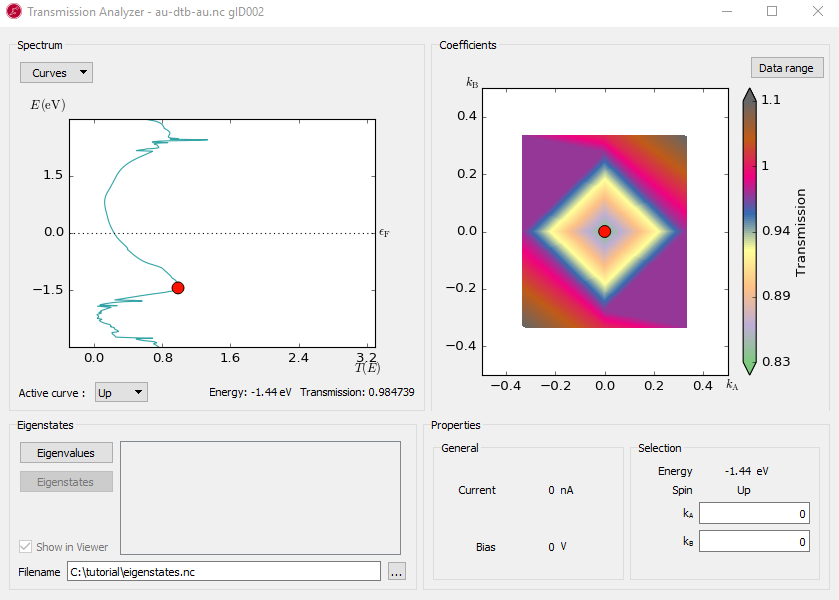There are three broad peaks, at around -3, -1.4 and 2.5 eV. Also note the narrow peak around 2.4 eV which rises above T(E)=1. In the following you will investigate the origin of these peaks.

Next select the DeviceDensityOfStates objectand click the Show 2D Plot... button to open the PDDOS analyzer window.To merge both PDDOS lines into one, click the Options button on the upper left corner and turning off flipping.Select all carbon and hydrogen atoms by enclosing them with a rectangle using left mouse button (or select the individual atoms with Ctrl left-click).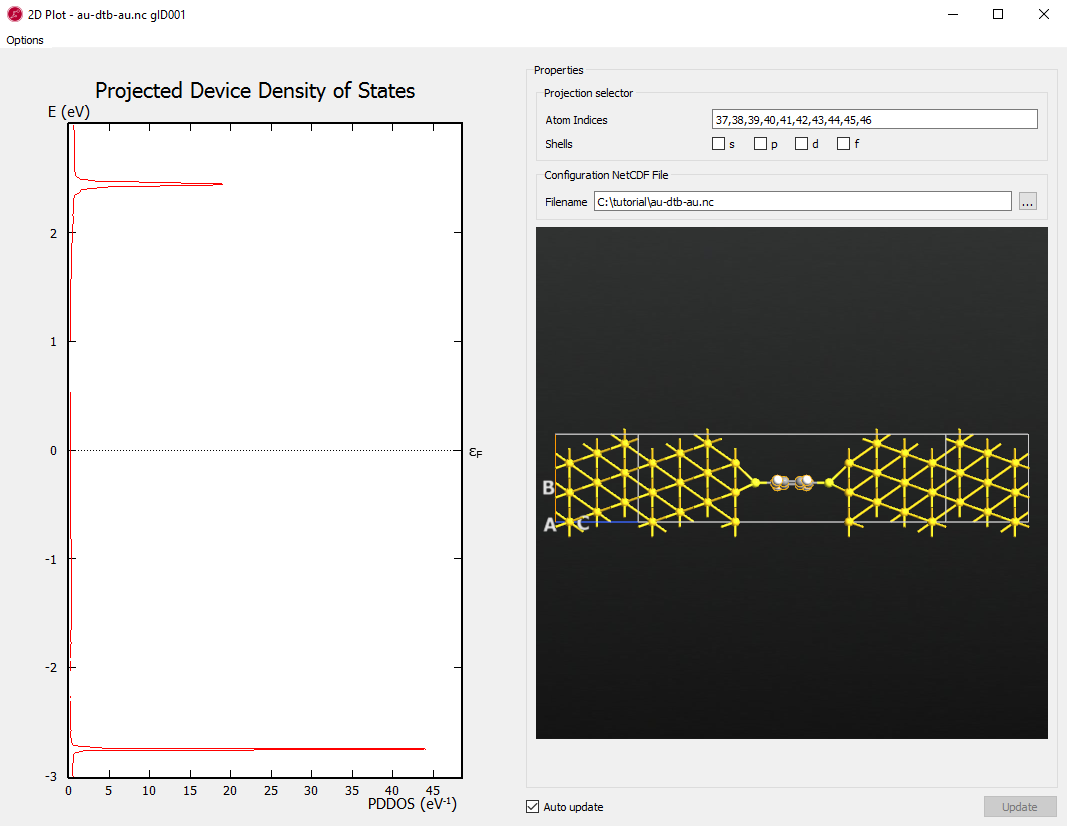Tip

Copy the Atom Indices here as you will need to input the indices in the next step.

Note

The similarity in the peak structure of the PDDOS and the transmission spectrum indicates a clear correspondence between the energy levels on the phenylene ring and the transmission spectrum.

### The Molecular Projected Self-Consistent Hamiltonian (MPSH)¶

To understand the energy levels of the phenylene ring in the presence of the surrounding electrodes, you will now calculated the MPSH states. The MPSH states are obtained by diagonalizing the molecular part of the full self-consistent Hamiltonian [STB+03].

Open the Script Generatortool and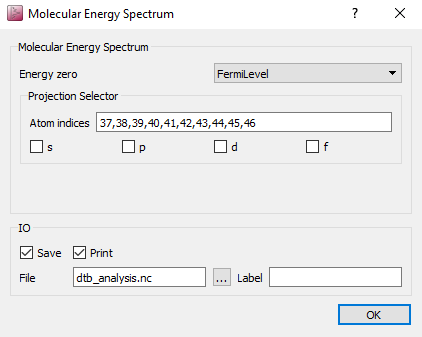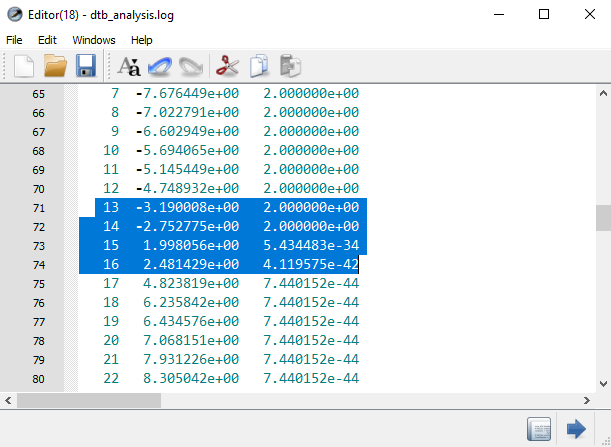To calculate the eigenstates of the MPSH levels, reopen the last Script Generator(it is available from the windows menu at the top of each QuantumATK window, if you didn’t close it).

• Change the name of the output file to molecular_eigenstate.hdf5.
• Add four Analysis ‣ EigenStateblocks.
• Open each EigenState script block and select projection on carbon and hydrogen Set the Quantum number to 13, 14, 15, 16 in each respective block.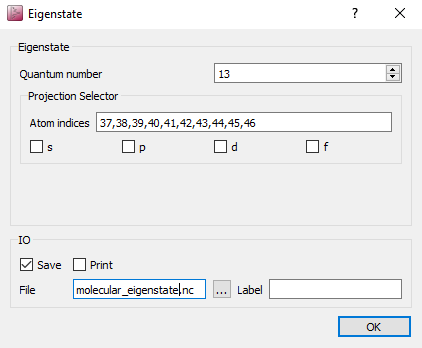When the calculation is done, the molecular_eigenstate.hdf5 file should already be ticked under the Project Files, so its objects will appear on the LabFloor. Select the first eigenstate object. Click the button Viewer in the tool bar on the right-hand side and select Isosurface. In the Viewer, open the Properties menu, select the Isosurface tab and do the following settings.

• Set the Isovalue to 0.16.
• Set the Color map to BlueRed
• Tick $$\pm$$ IsovalueTo visualize the eigenstate together with geometry, drag and drop the file builder_device.py onto the Viewer. Do the same to the other eigenstates and you will get the figures below: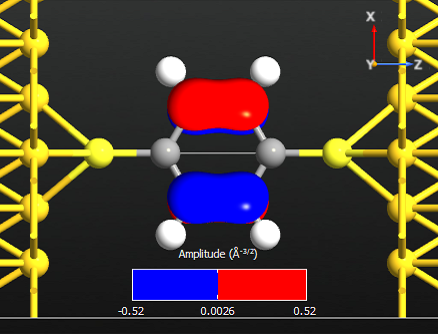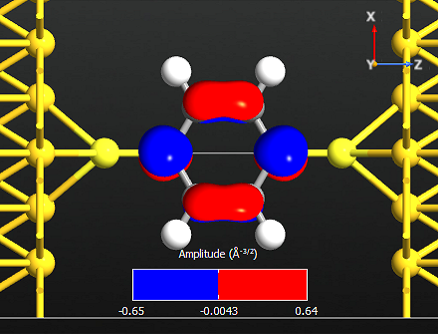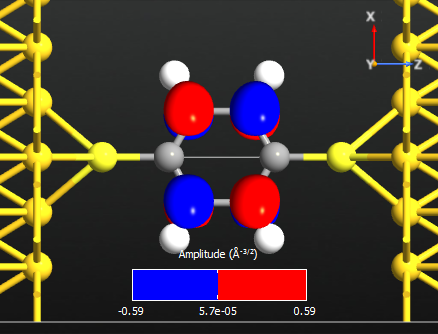Note that all the states are anti-symmetric in the plane of the benzene ring. This means that they are the $$\pi$$-orbitals of the benzene ring, i.e. they are linear combinations of the p-orbitals on each carbon atom which are perpendicular to the plane of the molecule. There are 6 such p-orbitals which means that there are 6 $$\pi$$-orbitals.

By visually inspecting the symmetry of the different eigenstates the remaining two $$\pi$$-orbitals are identified as state 10 and 18. Use Editor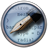to open the previous script, delete two Eigenstate blocks and change the quantum_number in the remaining two Eigenstate blocks to be 10 and 18 respectively. These are visualized below with an iso-value of 0.16.

Note

There are 6 electrons in the $$\pi$$-band, corresponding to that lowest 3 states are occupied. We may therefore denote state 14 the HOMO and state 15 the LUMO.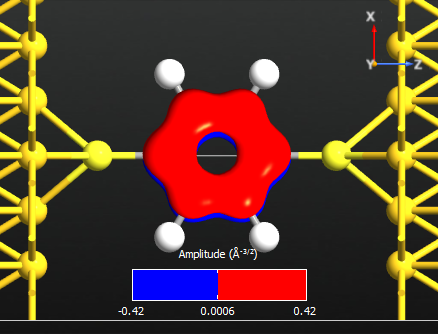### Transmission eigenvalues and eigenstates¶

In this chapter you will analyze the transmission eigenstates at the peak positions in the transmission spectrum, i.e. at energies -3, -1.5 and 2.4 eV.The transmission eigenvalues are obtained by diagonalizing the transmission matrix. The number of eigenvalues indicates the number of individual channels through the molecule, and the eigenvalue shows the strength of each channel. The eigenvalues are the true transmission probabilities, and thus lie in the interval [0,1]. If several channels are available at a particular energy, their sum - and hence the transmission coefficient at this energy - may however be larger than 1.

Most of the eigenvalues are very small and can be neglected. The two largest eigenvalues of each energy are the most important ones.

Note

At the first two energies there is only one dominating eigenstate, while at the last energy there are two dominating eigenvalues. The reason that they do not sum up to the total transmission spectrum T(E) will be discussed in the next section.

The next step is to visualize the transmission eigenstates for the first two peak energies, where there is only one significant eigenvalue.

The objects will again be saved in the file dtb_analysis.hdf5 and can be visualized using the same approach as for the eigenstates. Below are shown isosurfaces of the two transmission eigenstates.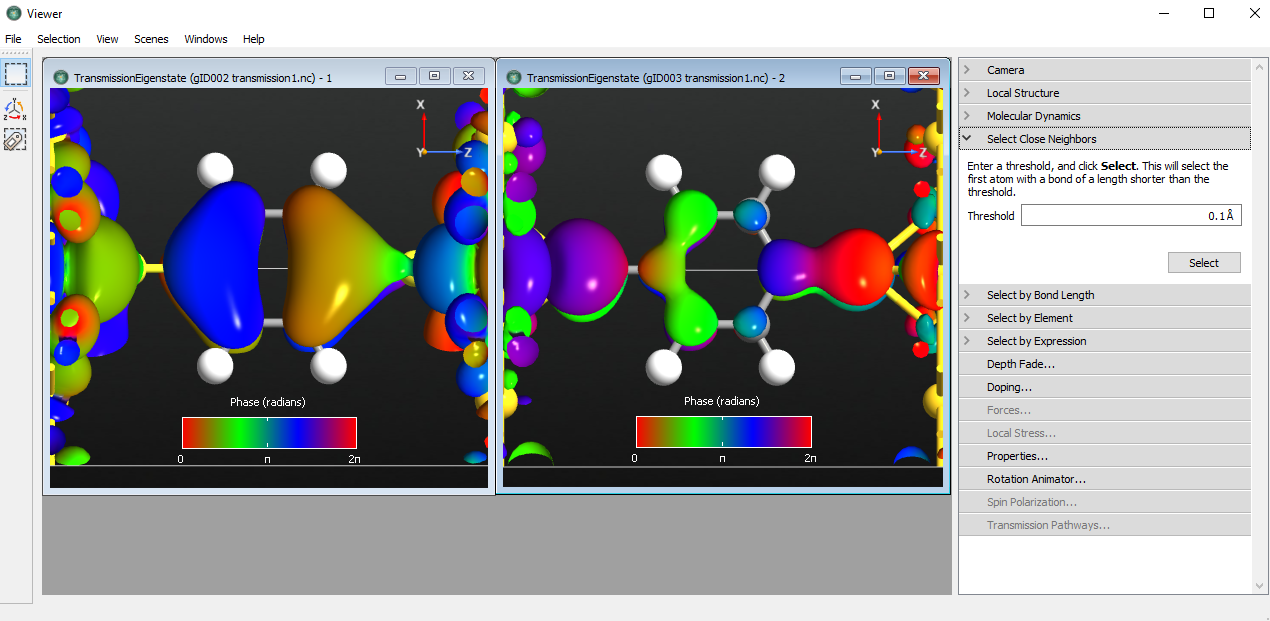Fig. 83 Transmission eigenstates of energy -3 and -1.5 eV

Tip

The transmission eigenstate is a complex wave function. The isosurface shows the absolute value of the wave function while the color of the isosurface indicates the phase. Since the phase is periodic, it is best to use a periodic color map.

The transmission eigenstate at -3.0 eV has a clear resemblance with MPSH state 13, i.e. the HOMO-1 state. The transmission eigenstate at -1.5 eV is also a $$\pi$$-state.

### k-dependent transmission and eigenchannels¶

You will now analyze the transmission at 2.4 eV. Previously, you found that there is an important transmission eigenstate at this energy with transmission probability 0.618 which is different from the value 1.45 obtained from inspecting Fig. 82. This is because Fig. 82 shows the k-point averaged transmission. You will now calculate the k-dependent transmission coefficients at this energy.

When the calculation has finished you can visualize the k-dependent using Transmission Analyzer.Fig. 84 The k-dependence of transmission at energy point 2.4eV

The contour plot of the k-dependent transmission above has a pronounced peak at $$(k_A,k_B)=(0.0,0.38)$$, with a transmission coefficient of around 1. In the computation of the transmission spectrum earlier, we did not have a dense enough k-point sampling, and hence the transmission was overestimated, i.e. we obtained 1.45 while the 21x21 mesh gives around 1.1.

Attention

This shows the importance of carefully checking the convergence of the results in the k-point sampling of the transmission spectrum. There is no reason to assume that the same number of points used to get a correct electron density (i.e. the k-points used in the self-consistent calculation) also give an accurate transmission.

You will now calculate the transmission eigenstates at the k-point with the strongest transmission. We can choose either point (0, -0.38) or (0, 0.38), they are equivalent by time-reversal symmetry.

• Click the point (0, -0.38) or (0, 0.38) on the transmission contour plot.
• Click Eigenvalues in the Eigenstates block on the lower left side. You will get a list of eigenvalues.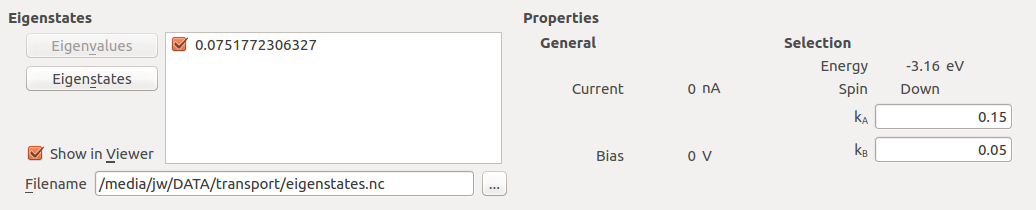• Select the first eigenvalue only, and press the button Eigenstates. The first eigenstate will be calculated and the Viewer window will pop up with a figure. Tune the isovalue in Properties to display the eigenvalue image nicely.Fig. 85 The first eigenstate contributes to the transmission at energy point 2.4 eV.

### Projected local DOS¶

In this section you will calculate the local device density of states throughout the device, projected onto the real-space transport direction (the Z-axis).

Execute the script – the calculations may take up to an hour to run. The PLDOS item will be added to the QuantumATK LabFloor. Plot the results using the Projected Local Density of States plugin in the right-hand plugins menu. Adjust the data range to get a plot like the one shown below.Fig. 86 Local device density of states projected onto the device Z-axis.

The PLDOS shows a high density in the metallic gold electrodes (pink regions), while the black color in the middle corresponds to vanishing DOS in the vacuum gap between the electrodes. In the middle, where the molecule is, there are three peaks in the local DOS as a function of energy, positioned around -3, -1.4, and +2.5 eV, in agreement with the peaks in the transmission spectrum.

## I-V characteristics¶

In this chapter you will calculate the I-V characteristics of the Au-DTB-Au molecular device, and analyze the device for an applied bias of 1.6 V.

### Setting up the calculation¶

Open a new Script Generatorwindow, and do the following:

Edit the Analysis from File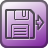block:

• Set HDF5 file to au-dtb-au.hdf5, and use gID000 to restart from the zero-bias calculation.

Also edit the IVCurveblock:

• Set the voltage bias range to end at V0 = -2V and V1 = 2 eV.
• Set the number of voltage points to 11.
• Set the energy range as (-3,3).
• Use 3x3 transverse k-points.
• Choose the Krylov self-energy calculator (for speed).The script is now done. Save it as iv-curve.py and execute it using the Job Manageror from the command line. This calculation will take some time, because a self-consistent SCF calculation and a transmission spectrum analysis must be done at each bias point. It takes six hours to finish in a 12-core machine.

### IV characteristic analyzation¶

Once the calculation is done, the file IV-curve.hdf5 appears in the folder. Eleven new output files are also created, prefixed ivcurve; they contain the converged SCF state at each bias point.NoteThe IVCurve object contains the calculated I–V curve, including the transmission spectra computed at each bias voltage.

Select the IV-curve and click the IV-Plot analysis tool in the right-hand plugins bar. The I–V curve is plotted in the window that pops up.

Check the box Additional plots. Three additional panels appear, plotting the differential conductance (dI/dV), the calculated transmission spectra, and the spectral current.Fig. 87 Each transmission spectrum and spectral current correspond to a specific bias voltage point. The energy range corresponding to the bias window is indicated in blue. By moving the mouse cursor to one of the marker points on either the IV or dI/dV plot, the corresponding transmission spectrum and spectral current will be highlighted.

Attention

Pay special attention to the spectral current: All possible contributions to the total current must be included inside the specified energy window, in order to get accurate $$I(V)$$ points. If this is not the case, you should consider redoing the analysis using a wider energy window.

Note

The peak in the dI/dV around 1.6 V arises from a resonance in the transmission spectrum entering the bias window at this voltage.

### PLDOS at 1.6 V bias¶

To investigate in details the electronic features of the molecular device under bias of 1.6V, you should compute the projected local density of states, just like you did above for zero bias, in section Projected local DOS.

Run the script and use agin the Projected Local Density of States plugin to visualize the results.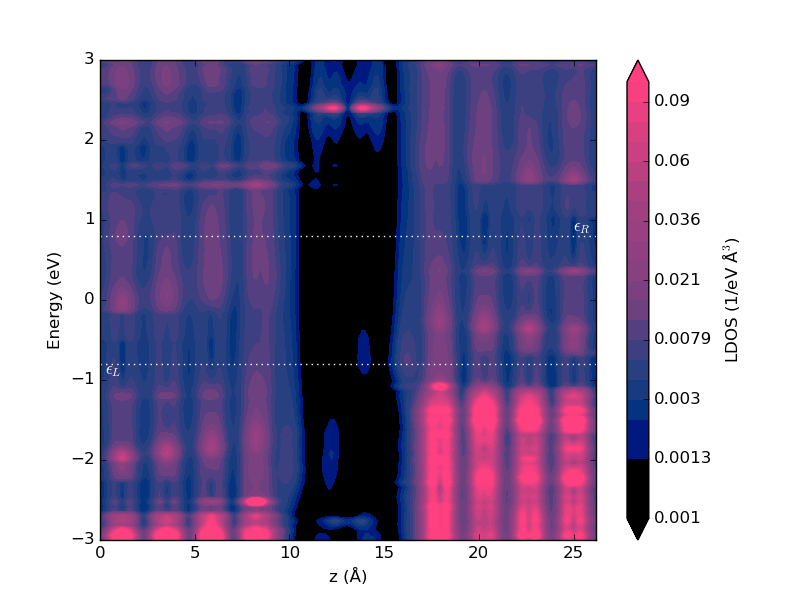Fig. 88 Projected local device DOS at 1.6 V applied bias.

Compared with Fig. 88, the resonance at -1.5 eV has split into two resonances, at -0.8 and -2 eV, as we saw already in the transmission spectrum above. We also see that the resonance at -0.8 is located towards the right electrode, while the resonance at -2 eV is located towards the left electrode. The splitting arises from the drop in the electrostatic potential through the molecule (due to the applied bias), as will be investigated in the next section.

### Calculating the voltage drop¶

You will now calculate the voltage drop and induced density for an applied bias of 1.6 V. At first, we will calculate the ElectrostaticDifferencePotential and ElectronDifferenceDensity between 1.6 V and 0 V.Repeat the above recipe to calculate the ElectrostaticDifferencePotential and ElectronDifferenceDensity at 0 V. Finally, you will have the files voltage_drop_16V.hdf5 and voltage_drop_0V.hdf5 under Project files.

To calculate the voltage drop using the ElectrostaticDifferencePotential and ElectronDifferenceDensity at 1.6 V and 0 V, we use GridOperations in the right-hand panel bar.

• Select the two objects by holding the ctrl key while clicking on each $$\delta V_E(\mathbf{r})$$ icon in the voltage_drop_16V and voltage_drop_0V files on the LabFloor.
• Open the GridOperations widget.
• Make a function such as A1 - B1.
• Save as EDP.hdf5.Repeat the above process for the density to get the voltage-induced density.You should now have the calculated grids in the files EDP.hdf5 and EDD.hdf5. To visualize the ElectrostaticDifferencePotential and ElectronDifferenceDensity induced by the 1.6 voltage, do the following:

• Select the file EDP.hdf5 and open the Viewer in the panel.
• Change the plot type in the grid visualization selection to the CutPlanes.
• Drag the builder_device.py on the window.
• Next, select the file EDD.hdf5 and drag into the same window.
• Keep the default, Isosurface for the ElectronDifferenceDensity.

You can tune the CutPlanes and Isosurface in the properties. In the case of CutPlanes, you can adjust the 3D and position of the CutPlane.If you apply the settings shown above, you should now have the following figure: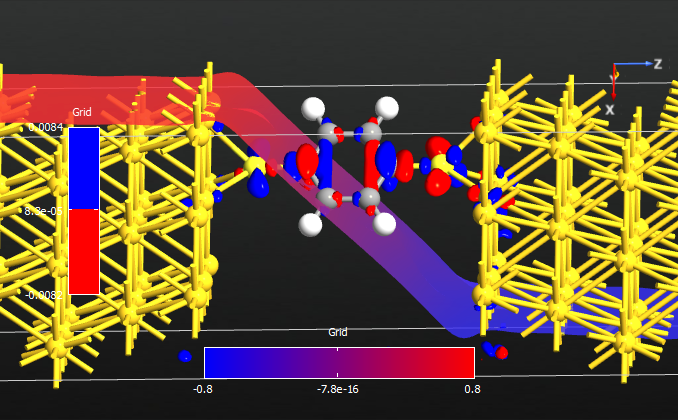Fig. 89 Electrostatic potential drop and electron density difference induced by a bias of 1.6V.

It is useful to make a 1D plot of the voltage drop. To this end, select the $$f(x)$$ of the EDP in the LabFloor and click the 1D Projector in the right hand panel bar. Then change the projection type to Average and click Add line.Fig. 90 Voltage drop along a line through the molecular axis.

In the case of 1D projection plot, c-axis shows the relative length between 0 and 1 corresponding to the real z distance.

Note

Both Fig. 89 and Fig. 90 show the total voltage drop of 1.6 V across the device. It is also clear from both figures that most of the voltage drop takes place around the molecule, not in the electrodes. A drop in voltage corresponds to a quantum resistance, and this explains the observed finite-bias splitting of the zero-bias resonance.